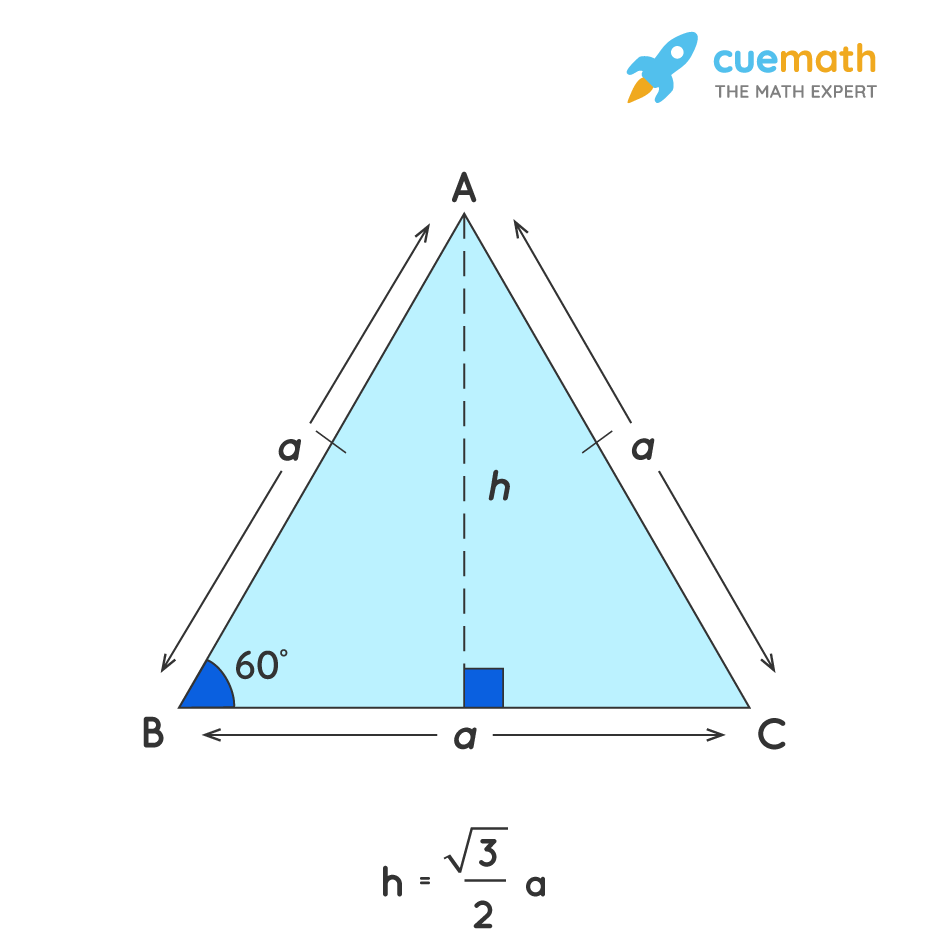# The Side Length of an Equilateral Triangle is 6 cm. What is The Height of The Triangle?

## Question: The side length of an equilateral triangle is 6 cm. What is the height of the triangle?

An equilateral triangle is a regular polygon with 3 equal sides.

## Answer: The height of the given equilateral triangle of side 6cm is h = 3√3 cm.

Let us find the height of the given equilateral triangle.

## Explanation:

Height of an Equilateral Triangle, h = (√3/2)aHere, the side of the given equilateral triangle is 6 cm.

a = 6cm

h = (√3/2)a = (√3/2) × (6) = 3√3 cm